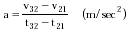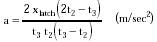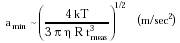Nanomedicine, Volume I: Basic Capabilities

Robert A. Freitas Jr., Nanomedicine, Volume I: Basic Capabilities, Landes Bioscience, Georgetown, TX, 1999

4.3.3.2 Displacement Accelerometers

Formed elements in the human bloodstream (e.g., red cells, white cells, platelets) typically are buffeted by much smaller accelerations. For example at 310 K, instantaneous random thermal accelerations of athermal ~ 500 g's are experienced by a 0.2-micron virus particle, ~0.05 g's by a 2-micron platelet, ~10-4 g's by a 10-micron neutrophil -- and ~0.4 g's by a 1 micron3 spherical nanorobot. Force-based sensors cannot easily detect such low accelerations, since the smaller the sensor, the larger the amin for a given SNR (Eqn. 4.11). An alternative approach uses displacement sensors to determine an object's velocity twice in rapid sequence, allowing acceleration to be computed from the difference.

Consider a constantly accelerating object that triggers three clocking latches at positions x1, x2, and x3, in sequence. Time and position of the object are measured as (x1, t1), (x2, t2), and (x3, t3). The measured constant acceleration is then given by{Eqn. 4.12}

where v32 = (x3 - x2) / (t3 - t2), v21 = (x2 - x1) / (t2 - t1), t32 = (t3 + t2) / 2 and t21 = (t2 + t1) / 2. If adjacent latches are an equal distance xlatch apart so that xlatch = (x3 - x2) = (x2 - x1), and taking t1 = 0, then Eqn. 4.12 reduces to{Eqn. 4.13}

Assume that a slowly accelerating external object passes three clocking latches that are fixed to the exterior of a nanorobot of radius R. Measurements of the object's velocity incur an unavoidable error due to nanorobot thermal motion. The object is observed to pass between latch pairs during a measurement time ~tmeas. During this same interval, from Eqn. 3.1 the nanorobot translates a distance DX = (kT tmeas/ 3 p h R)1/2, incurring a maximum velocity measurement error of ~DX / tmeas, and thus from Eqn. 4.12 a maximum acceleration measurement error of ~2 DX / tmeas2 if (t3 - t2) ~ (t2 - t1) ~ tmeas. Thus the minimum detectable acceleration is{Eqn. 4.14}

Taking T = 310 K, h = 1.1 x 10-3 kg/m-sec and R = 1 micron, then amin ~ 10-7 g's for tmeas = 1 sec; amin ~ 0.004 g's for a more reasonable tmeas = 10-3 sec measurement time in the in vivo environment. Onboard reference oscillators with the requisite accuracy are described in Section 10.1.

Last updated on 17 February 2003